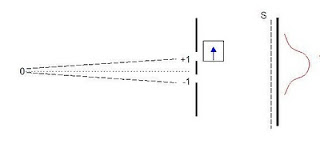## Wednesday, February 02, 2011

### Two-slit experiment

A second look at the two-slit experiment:

In Quantum Mechanics, two states can evolve, and we write this process as,

|A> → |A'>
|B> → |B'>

By the principle of linear superposition and unitarity, the superposition of these two states will also evolve as,

|A> + |B> → |A'> + |B'>

We label the position from which the electrons pass through as such: where they leave is 0; the slits are labelled +1 and –1; and the screen, S. The states representing the electron passing in one slit are |+1> and going through the second slit, |-1>. When the electrons leave position 0, from the symmetry of the setup, we can say that they arrive at position +1 and –1 with equal probability. So we write,

|0> → |+1> + |-1>

When an electron has arrived at +1 or -1, what happens after that when they hit the screen? Experiments show that they can land on any of the points on the screen, so we write,

|+1> → Σ Ψn|n>
|-1> → Σ Φn|n>Fig. 1

The whole process can be described as,

|0> → |+1> + |-1> → Σ Ψn|n> + Σ Φn|n>

= Σ(Ψn + Φn) |n>

The probability that an electron will arrive at the mth point on the screen is,

Pm = (Ψ*m< m| + Φ*m< m|)(Ψm|m> + Φm|m>)
= Ψ*mΨm< m|m> + Φ*mΦm< m|m> + Ψ*mΦm< m|m> + Φ*mΨm< m|m>

Using < m|m> = 1

Pm = Ψ*mΨm + Φ*mΦm + Ψ*mΦm + Φ*mΨm

The first term Ψ*mΨm represents the probability if only the first slit was open. Similarly, the second term Φ*mΦm represents the probability if only the second slit was open. Classically, we should get the sum of these two terms if both slits were open. But we do not observe that. The interesting aspect of this result from quantum physics is that we get two extra terms, Ψ*mΦm and Φ*mΨm, that correctly explains the interference pattern of the double-slit experiment (see fig. 1). Another major difference between classical physics and quantum physics is that in the first, probabilities add, while in the second, the amplitudes add and then we square the amplitudes to get the probabilities.

The Act of Measuring and Entanglement

Suppose we want to know through which slit the electron has passed. This can be done by inserting a detector at position +1. Furthermore, we prepare the electron at position 0 with a down spin. When it passes through +1, its spin is flipped to an up spin, and when it passes through position –1, nothing happens to the electron (see fig. 2). With this setup, we know when the electron has passed through slit labeled +1.Fig. 2

We need two labels for the states: one for position, and the other for the spin. We describe the process as,

|0,d> → |+1,u> + |-1,d> → Σ Ψn |n,u> + Σ Φn |n,d>

Due to the presence of the detector, the electrons are entangled through their spins: one is up, the other is down. Note: entanglement means that the system is prepared in such that if we know the spin of one of the particles, we automatically know the spin of the other particle. Again to calculate the probability of finding the electron at the mth position, we square the amplitudes, or multiply the amplitude with their complex conjugate.

Pm = (Ψ*m < m,u| + Φ*m< m,d|)(Ψm|m,u> + Φm|m,d>)
= Ψ*mΨm< m,u|m,u> + Φ*mΦm < m,d|m,d>
+ Ψ*mΦm< m,u|m,d> + Φ*mΨm< m,d|m,u>

Note for the last two terms, because the electrons have opposite spins, the up and down vector states are now orthogonal to each other.

< m,d|m,d> = < m,u|m,u> = 1
< m,u|m,d> = < m,d|m,u> = 0

Therefore,

Pm = Ψ*mΨm + Φ*mΦm

This result is completely different from the previous result. We see now that the very act of detecting the spin of one electron, that is, making some sort of measurement, destroys the interference pattern. This is another markedly difference between a classical system, in which we can always make a measurement without disturbing it, and a quantum system, in which a measurement entails disturbing the system and getting a different result.